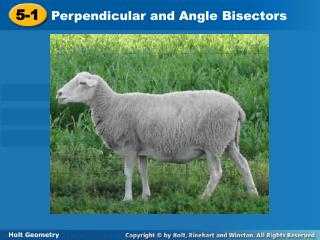DownloadDownload Presentation5-1

5-1

Télécharger la présentation5-1

- - - - - - - - - - - - - - - - - - - - - - - - - - - E N D - - - - - - - - - - - - - - - - - - - - - - - - - - -
Presentation Transcript

1. 5-1 Perpendicular and Angle Bisectors Holt Geometry

2. Warm Up Construct each of the following. 1.A perpendicular bisector. 2. An angle bisector. 3. Find the midpoint and slope of the segment (2, 8) and (–4, 6).

3. Objectives Prove and apply theorems about perpendicular bisectors. Prove and apply theorems about angle bisectors. Q: What do you get if you divide the circumference of a jack-o-lantern by its diameter? A: Pumpkin Pi!

4. Vocabulary equidistant locus

5. When a point is the same distance from two or more objects, the point is said to be equidistantfrom the objects. Triangle congruence theorems can be used to prove theorems about equidistant points.

6. A locus is a set of points that satisfies a given condition. The perpendicular bisector of a segment can be defined as the locus of points in a plane that are equidistant from the endpoints of the segment.

7. Example 1A: Applying the Perpendicular Bisector Theorem and Its Converse Find each measure. MN MN = LN  Bisector Thm. MN = 2.6 Substitute 2.6 for LN.

8. Example 1C: Applying the Perpendicular Bisector Theorem and Its Converse Find each measure. TU

9. Remember that the distance between a point and a line is the length of the perpendicular segment from the point to the line.

10. Based on these theorems, an angle bisector can be defined as the locus of all points in the interior of the angle that are equidistant from the sides of the angle.

11. Example 2A: Applying the Angle Bisector Theorem Find the measure. BC

12. Since EH = GH, and , bisects EFGby the Converse of the Angle Bisector Theorem. Example 2B: Applying the Angle Bisector Theorem Find the measure. mEFH, given that mEFG = 50°. Def. of  bisector Substitute 50° for mEFG.

13. Since, JM = LM, and , bisects JKL by the Converse of the Angle Bisector Theorem. Example 2C: Applying the Angle Bisector Theorem Find mMKL. mMKL = mJKM Def. of  bisector 3a + 20 = 2a + 26 Substitute the given values. a + 20 = 26 Subtract 2a from both sides. a= 6 Subtract 20 from both sides. So mMKL = [2(6) + 26]° = 38°

14. S is equidistant from each pair of suspension lines. What can you conclude about QS? Check It Out! Example 3

15. Step 1 Graph . The perpendicular bisector of is perpendicular to at its midpoint. Example 4: Writing Equations of Bisectors in the Coordinate Plane Write an equation in point-slope form for the perpendicular bisector of the segment with endpoints C(6, –5) and D(10, 1).

16. Step 2 Find the midpoint of . mdpt. of = Example 4 Continued Midpoint formula.

17. Since the slopes of perpendicular lines are opposite reciprocals, the slope of the perpendicular bisector is Example 4 Continued Step 3 Find the slope of the perpendicular bisector. Slope formula.

18. Step 4 Use point-slope form to write an equation. The perpendicular bisector of has slope and passes through (8, –2). Substitute –2 for y1, for m, and 8 for x1. Example 4 Continued y – y1 = m(x – x1) Point-slope form

19. Example 4 Continued

20. Use the diagram for Items 3–4. 3. Given that FH is the perpendicular bisector of EG, EF = 4y – 3, and FG = 6y – 37, find FG. 4. Given that EF = 10.6, EH = 4.3, and FG = 10.6, find EG. Lesson Quiz: Part I Use the diagram for Items 1–2. 1. Given that mABD = 16°, find mABC. 2. Given that mABD = (2x + 12)° and mCBD = (6x – 18)°, find mABC. 32° 54° 65 8.6

21. Lesson Quiz: Part II 5. Write an equation in point-slope form for the perpendicular bisector of the segment with endpoints X(7, 9) and Y(–3, 5) .